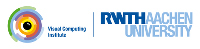OpenMesh
How to navigate on a mesh

# Introduction

In this tutorial you will learn how to navigate on a mesh using the OpenMesh library. In the previous chapter (see Mesh Iterators and Circulators) you have learned how to iterate over vertices, edges, halfedges and faces as well as circulate over certain structures such as 1-rings and many more. So in this tutorial we will focus on efficiently using the halfedge data structure and some very useful attributes such as the boundary flag. We assume that you already made yourself familiar with the halfedge structure which is used in OpenMesh. Further information on this topic can be found in The Halfedge Data Structure.

# Navigating over halfedges

So let's start with navigating over halfedges of a mesh. Assume we have the following mesh topology: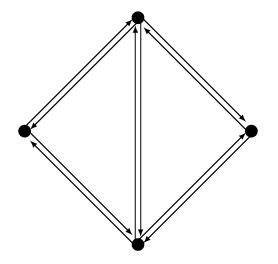We can now select an arbitrary halfedge of this mesh which then offers either one of two possible navigations:

• If the chosen halfedge lies at a boundary or in other words is not adjacent to a face, we can now navigate along the boundary (or hole) of our mesh by using `next_halfedge_handle()` or `prev_halfedge_handle()`: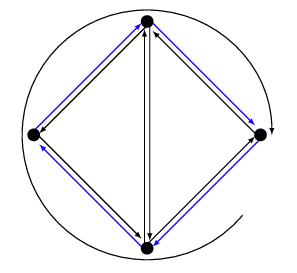• If the chosen halfedge is adjacent to a face, we can then navigate along all halfedges that are adjacent to this face. In other words we circulate around the inner halfedges of one face: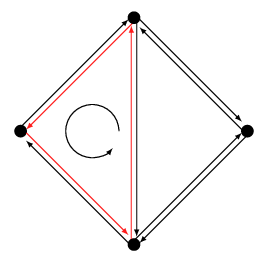In both cases the code would look something like the following example. Depending on whether the initial halfedge is adjacent to a face or not, we will either navigate on the boundary halfedges of our mesh or along the inner halfedges of a face:

[...]
TriMesh::HalfedgeHandle heh, heh_init;
// Get the halfedge handle assigned to vertex
heh = heh_init = mesh.halfedge_handle(vertex.handle());
// heh now holds the handle to the initial halfedge.
// We now get further on the boundary by requesting
// the next halfedge adjacent to the vertex heh
// points to...
heh = mesh.next_halfedge_handle(heh);
// We can do this as often as we want:
while(heh != heh_init) {
heh = mesh.next_halfedge_handle(heh);
}
[...]

# Mesh boundaries

As you have seen in the previous section, navigating along boundaries is very simple. In general OpenMesh also offers a boundary attribute for edges, vertices and faces. So testing i.e. whether a face is a boundary face is quite simple using OpenMesh::PolyConnectivity::is_boundary().

Note
You can iterate along boundaries by using the next_halfedge_handle(). If you are on a boundary, the next halfedge is guaranteed to be also a boundary halfedge.

So for each type we can make use of one of the following functions:

// Test if a halfedge lies at a boundary (is not adjacent to a face)
bool is_boundary (HalfedgeHandle _heh) const
// Test if an edge lies at a boundary
bool is_boundary (EdgeHandle _eh) const
// Test if a vertex is adjacent to a boundary edge
bool is_boundary (VertexHandle _vh) const
// Test if a face has at least one adjacent boundary edge.
// If _check_vertex=true, this function also tests if at least one
// of the adjacent vertices is a boundary vertex
bool is_boundary (FaceHandle _fh, bool _check_vertex=false) const

# Using incoming and outgoing halfedges

OpenMesh offers quite a lot of iterators and circulators to easily iterate over the structures of a mesh. A very helpful iterator is the OpenMesh::PolyConnectivity::VertexIHalfedgeIter or the OpenMesh::PolyConnectivity::VertexOHalfedgeIter which are used to iterate over all incoming/outgoing halfedges of a vertex. So, sticking to the illustration below, a OpenMesh::PolyConnectivity:V:ertexIHalfedgeIter for the lower most vertex would iterate over all incoming halfedges (blue), whereas the OpenMesh::PolyConnectivity::OpenMesh::PolyConnectivity::VertexOHalfedgeIter would iterate over all outgoing halfedges (red):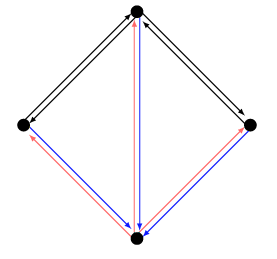A schematic code example of how to use the halfedge iterators as described above:

[...]
// Get some vertex handle
for(PolyMesh::VertexIHalfedgeIter vih_it = mesh.vih_iter(v); vih_it; ++vih_it) {
// Iterate over all incoming halfedges...
}
for(PolyMesh::VertexOHalfedgeIter voh_it = mesh.voh_iter(v); voh_it; ++voh_it) {
// Iterate over all outgoing halfedges...
}
[...]

# Using opposite halfedges

The halfedge structure splits every edge into two directional parts by creating two directed edges out of one undirected edge. So for every halfedge there exists its counterpart pointing in the opposite direction. OpenMesh allows to easily navigate through opposing halfedges via the function OpenMesh::Concepts::KernelT< FinalMeshItems >::opposite_halfedge_handle(). So in the illustration below `opposite_halfedge_handle()` for the blue halfedge would return the red halfedge: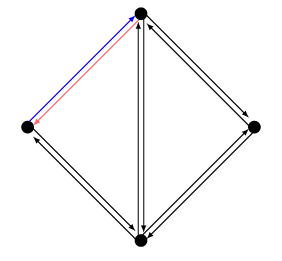Use this function as described in the example below:

// Get the halfedge handle of i.e. the halfedge
// that is associated to the first vertex
// of our set of vertices
PolyMesh::HalfedgeHandle heh = mesh.halfedge_handle(*(mesh.vertices_begin()));
// Now get the handle of its opposing halfedge
PolyMesh::HalfedgeHandle opposite_heh = mesh.opposite_halfedge_handle(heh);

There are also a few more functions that offer easy access to opposing structures:

// Get the face adjacent to the opposite halfedge
// Get the handle to the opposite halfedge
// Get the opposite vertex to the opposite halfedge
// Get the vertex assigned to the opposite halfedge

# Getting the to and from verticesIf you have an halfedge, you can get the to and the from handles of the adjacent vertices.

Note
The halfedges have a direction. Therefore the to vertex is the from vertex of the opposite halfedge and vice versa.
// Get the handle of the to vertex
// Get the handle of the from vertex

Project OpenMesh, ©  Computer Graphics Group, RWTH Aachen. Documentation generated using doxygen .